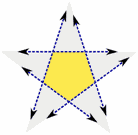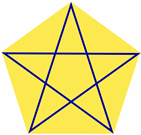# The PentagramThe Pentagram (or Pentangle) looks like a 5-pointed star.

You may think it has something to do with witchcraft, but in fact it is more famous as a magical symbol and is also a holy symbol in many religions.

In fact, this simple figure is quite amazing.

 Inside a Pentagram is a PentagonYou can make a pentagram by first drawing a pentagon, then extending the edges.Or by drawing lines from corner to corner inside a pentagon.

## Polygon

In fact a Pentagram is a special type of polygon called a "star polygon".## Ratios

The pentagram has a special number hidden inside called the Golden Ratio, which equals approximately 1.618

• a/b = 1.618...
• b/c = 1.618...
• c/d = 1.618...

When I drew this, I measured the 4 lengths and I got a=216, b=133, c=82, d=51. So let's check to see what the ratios are:

• 216/133 = 1.624...
• 133/82 = 1.622...
• 82/51 = 1.608...

If I had drawn and measured more accurately, I would have been even closer!

Why not have a go yourself:

• Draw a regular pentagram
• Measure the lengths
• Calculate the ratios

## Irregular Pentagram

This has all been about the regular pentagram (all sides and angles equal), but there are also irregular pentagrams.